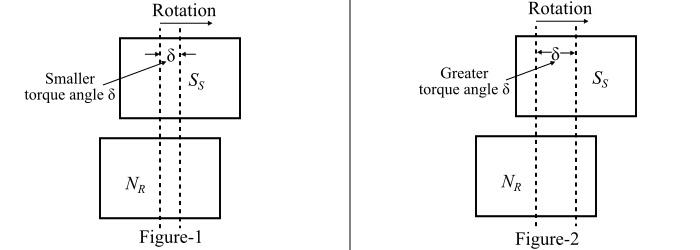# Effect of Load Change on a Synchronous Motor

In induction motors and DC motors, when the mechanical load attached to the shaft of the motor is increased, the speed of the motor decreases. The decrease in the speed reduces the back EMF so that additional current is drawn from the source to carry the increased load at a reduced speed. But, this action cannot take place in a synchronous motor, since it runs at a constant speed (i.e., synchronous speed) at all loads.For a synchronous motor, the armature current per phase is given by,

$$\mathrm{I_{a}=\frac{V-E_{f}}{Z_{s}}=\frac{E_{r}}{Z_{s}}\:\:\:\:\:\:...(1)}$$

When we apply the mechanical load to a synchronous motor, the rotor field poles slip back in space slightly behind the stator field poles (as shown in Figure-1), while the motor continuing to run at synchronous speed. In this process, the angular displacement between the stator and the rotor poles (called torque angle (δ) measured in electrical degrees) causes the phase of excitation voltage (Ef) to increase with respect to the supply voltage (V). This increases the resultant voltage (Er) in the armature winding of the motor. Hence, from Eqn. (1), it is clear that the armature current (Ia) increases to carry the load.

Also, the excitation voltage (Ef) is proportional to ωφ, thus, it only depends upon the field current and the speed of the motor. Since the motor is running with a constant speed and since the field current is also constant, the magnitude of the excitation voltage (Ef) remains constant with change in the load on the shaft of the motor.

If the load on the motor further increases, then the torque angle (δ) also increases, i.e., the rotor field poles lag behind the stator field poles by a greater angle (see Figure-2), but the motor continues to run at synchronous speed. The increase in the torque angle (δ) causes a greater phase displacement of the excitation voltage (Ef) with respect to the supply voltage (V). This increases the result voltage (Er) in the armature winding of the machine. Consequently, the armature current (Ia) increases to meet the load demand as seen from Eqn. (1).

Again, if the mechanical load on the shaft of the synchronous motor decreases, the torque angle (δ) also decreases. This causes the phase of excitation voltage(Ef) to decrease with respect to the supply voltage (V). As a result, the resultant voltage(Er) in the armature winding decreases and hence the armature current (Ia).

## Conclusion

When the load on a synchronous motor is increased, then

• The motor continues to run at synchronous speed

• The torque angle (δ) increases.

• The magnitude of the excitation voltage (Ef) remains constant

• The armature current (Ia) drawn from the supply increases.

When the load on a synchronous motor is decreased, then

• The motor continues to run at synchronous speed.

• The torque angle (Ia) decreases.

• The magnitude of the excitation voltage (Ef) remains constant.

• The armature current (Ia) drawn from the supply decreases.

Also, there is a limit to the mechanical load that can be applied to a synchronous motor. As the load on the motor is increased, the torque angle (δ) also increases till a stage is reached when the motor is pulled out of synchronism and the motor is stopped.